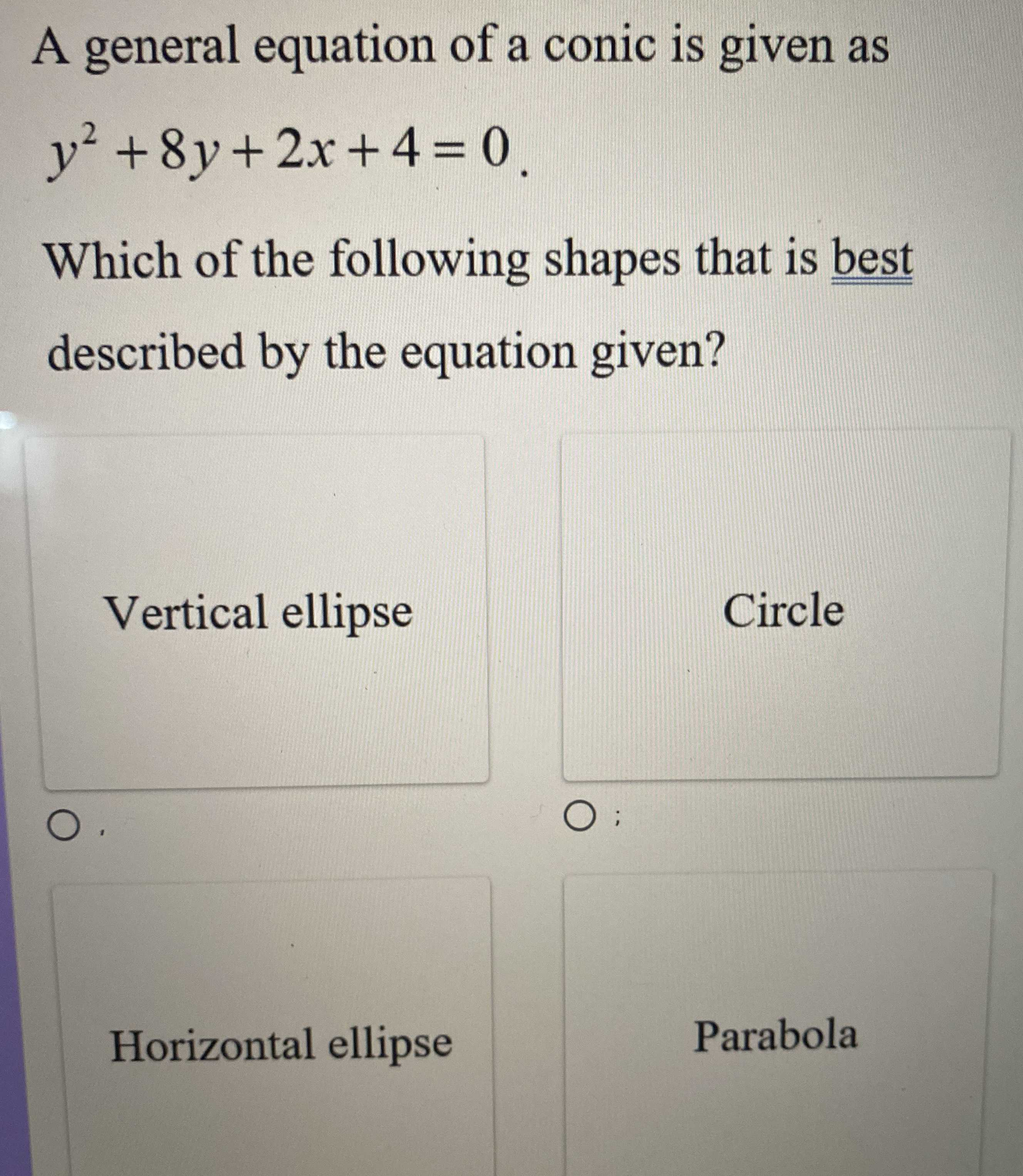### ¿Todavía tienes preguntas de matemáticas?

Pregunte a nuestros tutores expertos
Algebra
PreguntaA general equation of a conic is given as $$y ^ { 2 } + 8 y + 2 x + 4 = 0$$ .

Which of the following shapes that is best described by the equation given?